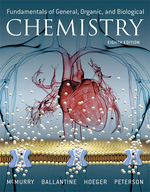×
Get Full Access to Fundamentals Of General, Organic, And Biological Chemistry (Mastering Chemistry) - 8 Edition - Chapter 9 - Problem 9.53
Get Full Access to Fundamentals Of General, Organic, And Biological Chemistry (Mastering Chemistry) - 8 Edition - Chapter 9 - Problem 9.53

×ISBN: 9780134015187 2044

## Solution for problem 9.53 Chapter 9

Fundamentals of General, Organic, and Biological Chemistry (Mastering Chemistry) | 8th Edition

• Textbook Solutions
• 2901 Step-by-step solutions solved by professors and subject experts
• Get 24/7 help from StudySoup virtual teaching assistantsFundamentals of General, Organic, and Biological Chemistry (Mastering Chemistry) | 8th Edition

4 5 1 353 Reviews
16
5
Problem 9.53

Over-the-counter hydrogen peroxide ($$\mathrm{H}_{2} \mathrm{O}_{2}$$ solutions are 3% 1m/v2. What is this concentration in moles per liter?

Text Transcription:

H_2O_2

Step-by-Step Solution:
Step 1 of 3

Step 2 of 3

Step 3 of 3

## Discover and learn what students are asking

Statistics: Informed Decisions Using Data : Introduction to the Practice of Statistics
?____________ are the characteristics of the individuals of the population being studied

Statistics: Informed Decisions Using Data : Measures of Position and Outliers
?Explain what each quartile represents.

Statistics: Informed Decisions Using Data : Inference about Two Population Standard Deviations
?In Problems 9–14, assume that the populations are normally distributed. Test the given hypothesis. 12. s1 6 s2 at the a = 0.05 level of signi

Statistics: Informed Decisions Using Data : Inference about the Difference between Two Medians: Dependent Samples
?Outpatient Treatment The median length of stay for substance-abuse outpatient treatment completers is 107 days for those referred by the criminal just

Unlock Textbook Solution